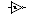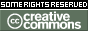DC Motors
How they work, in 4 parts -- 12 November, 2001

 History and background Principles of operation DC motor behavior Parameterizing performance

Parameterizing DC motor performance
Boiling things down to constants

As you tinker around with DC motors, you'll start to run across some interesting relationships. Namely you'll discover that torque and current are linearly proportional to each other, as are speed and voltage. Under a fixed load (torque), voltage and current will also be proportional to each other.

Digging into the math (and I'll spare you this), it turns out that the current a motor draws is ultimately determined by the torque the motor produces. The generated torque is dependent upon the current I, and factors determined by the materials and internal geometry of the motor. Since the construction of a finished motor will not (!) change during operation, a constant of proportionality between the motor current and the materials / geometry dependent factors can be calculated for a given motor. This constant, the torque constant Kt, describes the torque generated by the motor for a specific motor current:

Kt = T / I

Or to put it another way,

Current through motor = torque produced / torque constant

I (Amps) = Torque (oz-in) / Kt (oz-in/A) in imperial units

I (Amps) = Torque (N-m) / Kt (N-m/A) in SI units

Because of the interrelationship of torque, speed, current, and voltage, the constant current operation of a DC motor produces constant output torque regardless of speed. Given a constant load (i.e. torque) the speed of a motor is solely dependent on the voltage applied to the motor. For DC motors operated at a constant voltage, the speed and torque produced are inversely related (the higher the torque, the lower the speed of the motor).

We earlier saw that an EMF will be developed across a motor's brushes when its coil is rotated by an external torque -- the magnitude of this EMF is dependent upon materials / geometry factors, and upon the speed at which the coil is rotated. Once again, there is a constant of proportionality which describes the relationship between coil rotational speed and materials / geometry factors, commonly known as the back EMF constant (Ke). The back EMF constant is typically given in volts per unit of rotational speed (which in turn is generally expressed either in RPM or radians / second).

If one takes the reciprocal of the back EMF constant, the result is a proportionality constant which relates the voltage applied to the motor terminals to the rotational speed of the coil. This version of the motor constant is commonly known as the velocity constant, Kv. The velocity constant is given in units of rotational speed (again, either RPM or radians / second) per volt.

Since the motor construction does not change, regardless of what we're measuring, it turns out that these three constants (Kt, Ke, Kv) are all essentially the same number. The differences between the torque constant and the back EMF constant are simply a matter of the units used, while the velocity constant is simply a useful form of the back EMF constant.

If the torque constant is specified in N-m / A and the back EMF constant in V-sec / rad, then:

Kt = Ke = 1 / Kv

Those of us who live in the U.S., though, are stuck with using more colorful units. Commonly used units for small motors are oz-in for torque and RPM for rotational speed. Using these units of measure, torque constants are often given in oz-in / A, back EMF constants in mV / RPM, and velocity constants in RPM / V. In imperial units, the relationships between motor constants are then as follows:

With
Kt in units of oz-in / A
Ke in units of mV / RPM
Kv in units of RPM / volt

Then

Kt = 1352.4 / Kv

Ke= Kt / 1.3524

Ke = 1000 / Kv

Kv = 1000 / Ke

So what good is all this? It means that given a source of known rotational speed (an electric drill, or drill press if you have one), you can compute Ke for a given motor (clamp the motor shaft in the drill's jaws, measure the resulting open-circuit voltage, then do the math).

Ke, along with the above information will then give you Kt (so you can compute your motor's theoretical torque at any given current), and Kv (so you can compute your motor's maximum speed at any given voltage). If you can measure stall torque, you can then compute motor efficiency (measured torque expressed as a percentage of the theoretical torque).

Knowing all this, you can then pick the best motor for your BEAMbot's own specific needs.

 For more information Interested readers are urged to peruse the MicroMo pages on the development of electromotive force, on motor application considerations, on motor calculations, and on selecting a DC motor.

 History and background Principles of operation DC motor behavior Parameterizing performance

 HomeLegalitiesPage author: Eric Seale This page was last updated onThis work is licensed under a Creative Commons License.